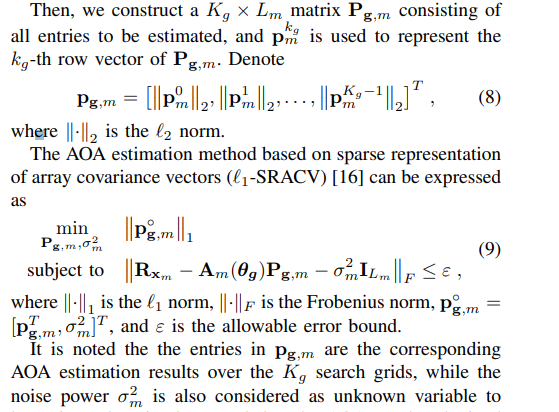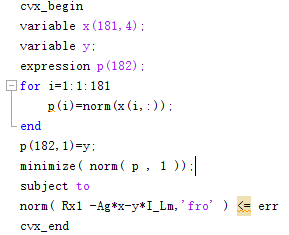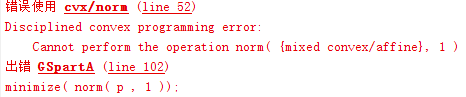# Minimize an expression which cause mixed convex/affine problem

I am new to CVX and I am working on understanding the Users’ Guide. I do have a problem that I am trying to minimize an expression made by following requirement:So I take Pg,m and sigma^2 as variables and p^og,m as the expression to achieve this optimization problem. I am pretty sure this problem is a CVX problem.I did the above attempt but results are as follows:I have been working on this problem for nearly a week but I cannot sort it out.
If possible, can someone tell me where am I wrong`norm(p,1)` is a norm of a norm, which is not allowed in CVX, because the argument of `norm` must be affine,

help cvx/norm

``````Disciplined convex programming information:
norm is convex, except when P<1, so an error will result if
these non-convex "norms" are used within CVX expressions. norm
is nonmonotonic, so its input must be affine.
``````

I believe `norm(p,1)` can be reformulated as `sum(p)`. Actually, the for loop for `p(i)` and `norm(p,1)` can be replaced by `sum(norms(x,[],2))`

help norms

norms Computation of multiple vector norms.
norms( X ) provides a means to compute the norms of multiple vectors
packed into a matrix or N-D array. This is useful for performing
max-of-norms or sum-of-norms calculations.

``````All of the vector norms, including the false "-inf" norm, supported
by NORM() have been implemented in the norms() command.
norms(X,P)           = sum(abs(X).^P).^(1/P)
norms(X)             = norms(X,2).
norms(X,inf)         = max(abs(X)).
norms(X,-inf)        = min(abs(X)).
If X is a vector, these computations are completely identical to
their NORM equivalents. If X is a matrix, a row vector is returned
of the norms of each column of X. If X is an N-D matrix, the norms
are computed along the first non-singleton dimension.

norms( X, [], DIM ) or norms( X, 2, DIM ) computes Euclidean norms
along the dimension DIM. norms( X, P, DIM ) computes its norms
along the dimension DIM.

Disciplined convex programming information:
norms is convex, except when P<1, so an error will result if these
non-convex "norms" are used within CVX expressions. norms is
nonmonotonic, so its input must be affine.``````

Thanks Mark,
I do really learn a lot from your suggestion which helps me sort out more optimization problems.